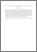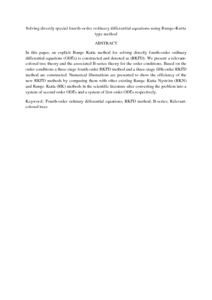# Solving directly special fourth-order ordinary differential equations using Runge-Kutta type method

## Citation

Hussain, Kasim and Ismail, Fudziah and Senu, Norazak (2016) Solving directly special fourth-order ordinary differential equations using Runge-Kutta type method. Journal of Computational and Applied Mathematics, 306. pp. 179-199. ISSN 0377-0427; ESSN: 1879-1778

## Abstract

In this paper, an explicit Runge–Kutta method for solving directly fourth-order ordinary differential equations (ODEs) is constructed and denoted as (RKFD). We present a relevant-colored tree theory and the associated B-series theory for the order conditions. Based on the order conditions a three-stage fourth-order RKFD method and a three-stage fifth-order RKFD method are constructed. Numerical illustrations are presented to show the efficiency of the new RKFD methods by comparing them with other existing Runge–Kutta Nyström (RKN) and Runge–Kutta (RK) methods in the scientific literature after converting the problem into a system of second order ODEs and a system of first order ODEs respectively.Preview
PDF
Solving directly special fourth-order ordinary differential equations using Runge–Kutta type method.pdfView Item